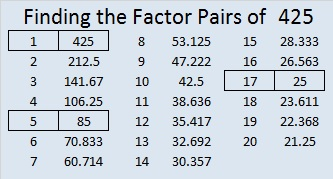# 425 and Level 6

425 ends in 25 so it can be divided evenly by 25. If I had \$4.25 all in quarters. How many quarters would I have? That’s the problem that I think of when I divide by 25. All of the factors of 425 are listed below the puzzle.

For some reason unknown to me, here in the United States, dates are ordered by month, date, and year. This rather illogical way of ordering allows us to say that today is 3-14-15, which are the first five digits of pi.  It could also be said that 3-14-15 at 9:26:53 gives the first ten digits of pi.

Logical or not, it is fun to declare today as Pi Day. Today’s puzzle celebrates those first five digits:Print the puzzles or type the factors on this excel file: 12 Factors 2015-03-09

• 425 is a composite number.
• Prime factorization: 425 = 5 x 5 x 17, which can be written 425 = (5^2) x 17
• The exponents in the prime factorization are 2 and 1. Adding one to each and multiplying we get (2 + 1)(1 + 1) = 3 x 2  = 6. Therefore 425 has exactly 6 factors.
• Factors of 425: 1, 5, 17, 25, 85, 425
• Factor pairs: 425 = 1 x 425, 5 x 85, or 17 x 25
• Taking the factor pair with the largest square number factor, we get √425 = (√25)(√17) = 5√17 ≈ 20.6155425 and all of it factors (except 1) are hypotenuses of primitive Pythagorean triples, so 425 is the hypotenuse of several triples:

• [87-416-425] and
• [297-304-425] are primitives
• [65-420-425] is [13-84-85] times 5
• [119-408-425] is [7-24-25] times 17
• [180-385-425] is [36-77-85] times 5
• [200-375-425] is [8-15-17] times 25
• [255-340-425] is [3-4-5] times 85This site uses Akismet to reduce spam. Learn how your comment data is processed.